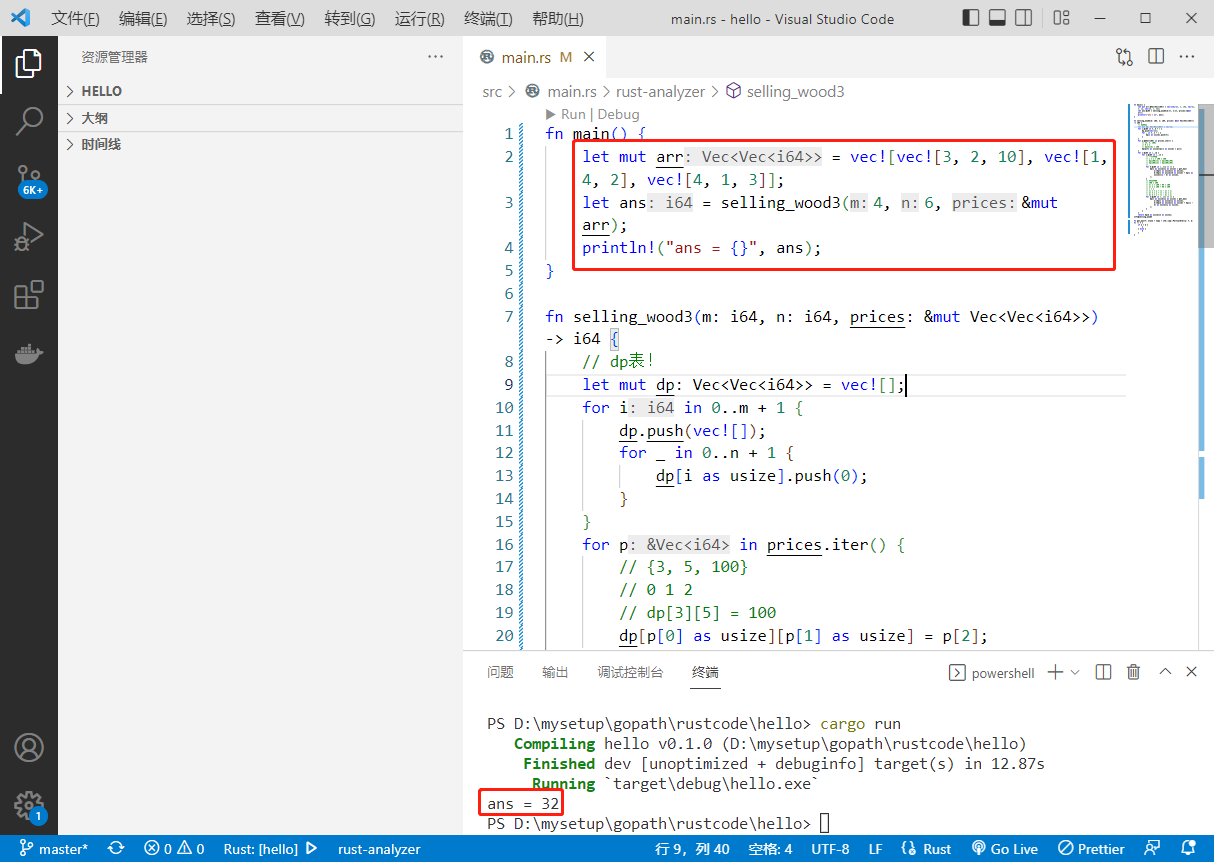# 2022-09-13：给你两个整数 m 和 n ，分别表示一块矩形木块的高和宽。 同时给你一个二维整数

2022-09-13：给你两个整数 m 和 n ，分别表示一块矩形木块的高和宽。

2)。

``````fn main() {
let mut arr = vec![vec![3, 2, 10], vec![1, 4, 2], vec![4, 1, 3]];
let ans = selling_wood3(4, 6, &mut arr);
println!("ans = {}", ans);
}

fn selling_wood3(m: i64, n: i64, prices: &mut Vec<Vec<i64>>) -> i64 {
// dp表！
let mut dp: Vec<Vec<i64>> = vec![];
for i in 0..m + 1 {
dp.push(vec![]);
for _ in 0..n + 1 {
dp[i as usize].push(0);
}
}
for p in prices.iter() {
// {3, 5, 100}
// 0 1 2
// dp = 100
dp[p as usize][p as usize] = p;
}
for i in 1..=m {
for j in 1..=n {
// 垂直分割
// i * j = 100 * 100
// dp + dp
// dp + dp
// ..
for k in 1..=(j >> 1) {
dp[i as usize][j as usize] = get_max(
dp[i as usize][j as usize],
dp[i as usize][k as usize] + dp[i as usize][(j - k) as usize],
);
}
// 水平分割
// 100 * 100
// 1) 1 * 100 + 99 * 100
// 1) 2 * 100 + 98 * 100
// i * j
// 1) 1 * j + (i - 1) * i;
// 2) 2 * j + (i - 2) * j;
// k) k * j + (i - k) * j;
for k in 1..=(i >> 1) {
dp[i as usize][j as usize] = get_max(
dp[i as usize][j as usize],
dp[k as usize][j as usize] + dp[(i - k) as usize][j as usize],
);
}
}
}
return dp[m as usize][n as usize];
}

fn get_max<T: Clone + Copy + std::cmp::PartialOrd>(a: T, b: T) -> T {
if a > b {
a
} else {
b
}
}
``````(=￣ω￣=)··· 暂无内容！

106

5

6

3